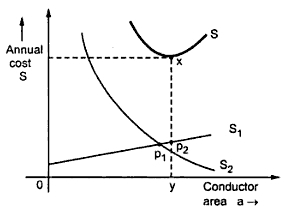### Modified Kelvin's Law

Due to limitations of applying Kelvin's law, some modification is necessary which was suggested by G. Kapp. While considering the cost of conductor, the cost associated with poles or towers, insulators, erection is not considered in case of overhead line while for underground cable, the cost of cable insulation and laying is not considered which forms appreciable part in the total cost in actual practice. With increase in conductor size, there is increase in mechanical stresses which needs stronger towers and insulators. This increases cost of labour for erection.
To account foe this costs and to get fair results practically, G. Kapp suggested to divide the initial investment into two parts i) one part which is independent of conductor size and ii) other part directly proportional to the conductor cross section.
The initial investment
S1 = K + K1 a
Annual cost of line, S1 = (Ko + K1 a) r if r is rate of interest and depreciation
Annual cost of power loss
S2 = K2/a
Total annual cost, S = S1 + S2
...                                 S = (K + K1 a) r + K2/a
For minimum cost,
dS/da = 0
Thus modified Kelvin's law suggests the most economical conductor size is one for which annual energy loss cost is equal to annual cost of interest and depreciation for that part of initial investment which is proportional to area of conductor.
The graphical representation of modified Kelvin's law is shown in the Fig. 1.Fig. 1
The cost S1 is represented by straight line. Its intercept on cost axis gives that part of annual cost which is not proportional to conductor area. The lost energy cost curve S2 is represented by rectangular hyperbola as before. The summation curve of these two costs is shown by S.
The lowermost point on total cost curve x gives minimum cost (xy) an economical conductor size (oy). The length P1 P2 is the cross section of the conductor cost which is not proportional to area of cross section of the conductor.